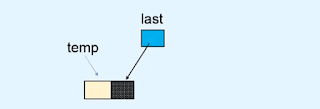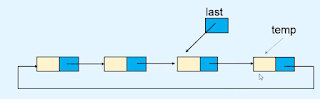To perform insertion operation in the Circular linked list we need a reference to the last node of the list. because if we use the reference of the first node to perform operations like insertion and deletion then we need more time to complete these operations.
so we use the reference of the last node in a circular linked list to perform all the insertion operations in constant time.

In this tutorial, we are going to learn how to
1. Insert a node at the beginning of the list.
2. Insert a node in an empty list.
3. Insert a node at the end of the list.

### Insert a node at the beginning of the Circular linked list.

first, we allocate a new node called temp.

### Insert a node in an empty circular linked list.

as we know an empty liked list doesn't have any node in it. so the last variable's value is None or Null. first, we allocate a new node called temp.and also it is the first and last node of the list. so we store the temp node is a reference into the linked part of the temp node. means temp node point himself.

### Insert a node at the end of the Circular linked list

first, we allocate a new node called temp.so now the temp node becomes the last node of the linked list. so we update the value of the last variable. because the last variable always stores the reference of the last node in a circular linked list.so after performing these steps now the new node is inserted at the end position of the linked list.

#### Python program for performing insertion in a circular linked list.

```class Node(object):

def __init__(self, value):
self.info = value

def __init__(self):
self.last = None
def display_list(self):

if self.last == None:
print("List is empty\n")

while True:
print(p.info, " ", end='')
break        print()

def insert_in_beginning(self, data):
temp = Node(data)

def insert_in_empty_list(self, data):
temp = Node(data)
self.last = temp

def insert_at_end(self,data):
temp = Node(data)
self.last = temp

def create_list(self):
n = int(input("Enter the number of nodes : "))
if n == 0:
return        data = int(input("Enter the element to be inserted : "))
self.insert_in_empty_list(data)

for i in range(n-1):
data = int(input("Enter the element to be inserted : "))
self.insert_at_end(data)

def insert_after(self, data, x):

while True:
if p.info == x:
break
if p == self.last.link and p.info != x:
print(x, " not present in the list")
else:
temp = Node(data)
if p == self.last:
self.last = temp

def delete_first_node(self):
if self.last is None:

def delete_last_node(self):
if self.last is None:
self.last = None            return
self.last = p

def delete_node(self,x):

if self.last is None:
return        if self.last.link == self.last and self.last.info == x:
self.last = None            return        if self.last.link.info == x:
return

else:
if self.last.info == x:
self.last = p

#################################################

list.create_list()

while True:
print("1. Display list")
print("2. Insert in empty list")
print("3. Insert a node in the beginning of the list")
print("4. Insert a node at the end of the list")
print("5. Insert a node after a specified node")
print("6. Delete first node")
print("7. Delete last node")
print("8. Delete any node")
print("9. Quite")

option = int(input("Enter your choice : "))

if option == 1:
list.display_list()
elif option == 2:
data = int(input("Enter the element to be inserted : "))
list.insert_in_empty_list(data)
elif option == 3:
data = int(input("Enter the element to be inserted : "))
list.insert_in_beginning(data)
elif option == 4:
data = int(input("Enter the element to be inserted : "))
list.insert_at_end(data)
elif option == 5:
data = int(input("Enter the element to be inserted : "))
x = int(input("Enter the element after which to insert : "))
list.insert_after(data,x)
elif option == 6:
list.delete_first_node()
elif option == 7:
list.delete_last_node()
elif option == 8:
data = int(input("Enter the element to be deleted : "))
list.delete_node(data)
elif option == 9:
break    else:
print("Wrong option")
print()```